# 深度神经网络 (第 III 部)。样品选择和降维

4 十月 2017, 07:50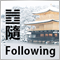0
3 531

### 1. 样品选择

• 一方面, 通过创建不会受到噪音很大影响的可靠分类算法来解决问题, 这是算法级别的途径。这种情况下每个算法都将是一个特定的, 非通用的解决方案。
• 另一方面, 数据级别的方法 (过滤器) 是尝试开发清洁数据的策略, 这将在分类器的训练之前进行。NoiseFiltersR 软件包使用第二种方法。这是因为它仅允许初步数据处理一次, 之后分类器可以根据需要进行多次更改。

```evalq({
#-----DT---------------------
out11 <- ORBoostFilter(Class~., data = DT\$train, N = 10, useDecisionStump = TRUE)
DT\$train_clean1 <- out11\$cleanData
#----------DTTanh.n------------------------
out1 <- ORBoostFilter(Class~., data = DTTanh.n\$train, N = 10, useDecisionStump = TRUE)
DTTanh.n\$train_clean1 <- out1\$cleanData
#-----------DTn--------------------------------
out12 <- ORBoostFilter(Class~., data = DTn\$train, N = 10, useDecisionStump = TRUE)
DTn\$train_clean1 <- out12\$cleanData
},
env)
#---Ris1-----------------
require(funModeling)
evalq({
par(mfrow = c(1,3))
par(las = 1)
boxplot(DT\$train_clean1 %>% select(-c(Data,Class)), horizontal = TRUE,
main = "DT\$train_clean1")
boxplot(DTn\$train_clean1 %>% select(-c(Data,Class)), horizontal = TRUE,
main = "DTn\$train_clean1")
boxplot(DTTanh.n\$train_clean1 %>% select(-c(Data,Class)), horizontal = TRUE,
main = "DTTanh.n\$train_clean1")
par(mfrow = c(1,1))
}, env)

```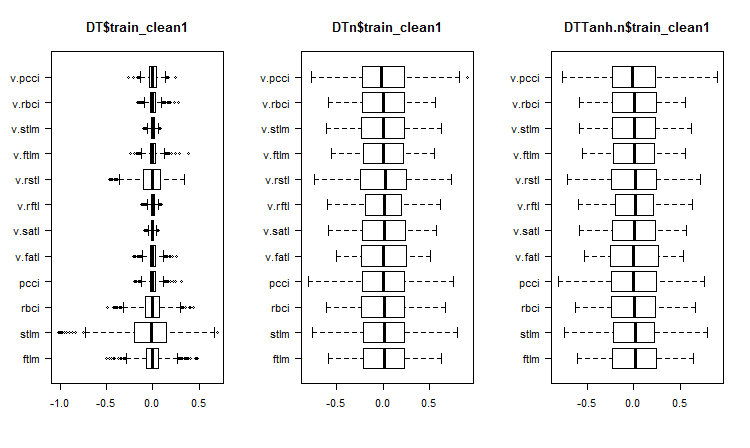```#----Ris2------------------
require(GGally)
evalq(ggpairs(DT\$train_clean1 %>% select(-Data),
columns = c(1:6, 13),
mapping = aes(color = Class),
title = "DT\$train_clean1/1"),
env)

```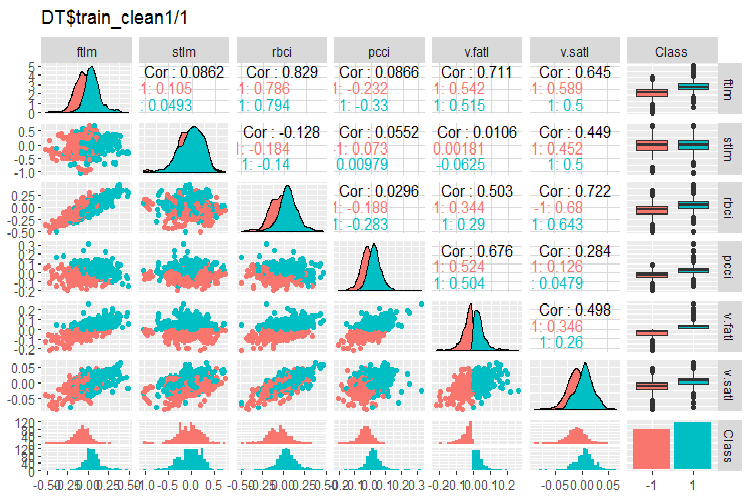```#-----Ris3---
evalq(ggpairs(DT\$train_clean1 %>% select(-Data),
columns = 7:13,
mapping = aes(color = Class),
title = "DT\$train_clean1/2"),
env)

```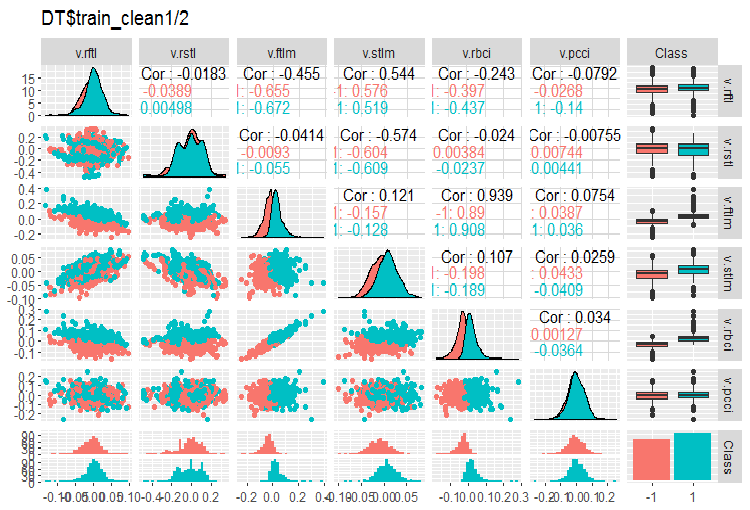```> env\$out11\$remIdx %>% length()
 658

```

1. 非常显着, 预测因子的分布通过目标变量进行划分。这有很大可能会显著提高我们正训练的模型的准确性。
2. 几乎所有的异常值已被移除。

```c(env\$out1\$remIdx %>% length(), env\$out12\$remIdx %>% length())
 652 653

```

```#----Ris4-----------------------
evalq(ggpairs(DTTanh.n\$train_clean1, columns = 1:13,
mapping = aes(color = Class),
upper = "blank",
title = "DTTanh.n\$train_clean_all"),
env)

```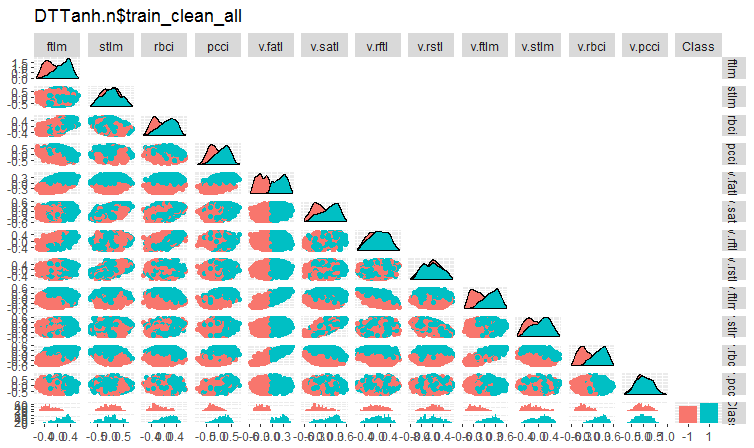```#-------ris5----------
require(GGally)
evalq(ggpairs(DTn\$train_clean1 %>% select(-Data),
columns = 1:13,
mapping = aes(color = Class),
upper = "blank",
title = "DTn\$train_clean1_all"),
env)

```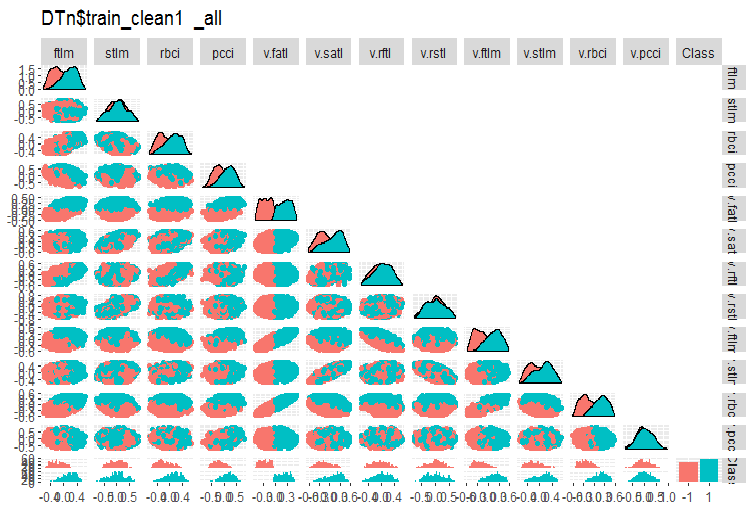```#--------Ris6---------------------------
require(smbinning)
par(mfrow = c(1,3))
evalq({
df <- renamepr(DT\$train_clean1) %>% targ.int
sumivt.dt = smbinning.sumiv(df = df, y = 'Cl')
smbinning.sumiv.plot(sumivt.dt, cex = 0.8)
rm(df)
},
env)
evalq({
df <- renamepr(DTTanh.n\$train_clean1) %>% targ.int
sumivt.tanh.n = smbinning.sumiv(df = df, y = 'Cl')
smbinning.sumiv.plot(sumivt.tanh.n, cex = 0.8)
rm(df)
},
env)
evalq({
df <- renamepr(DTn\$train_clean1) %>% targ.int
sumivt.dtn = smbinning.sumiv(df = df, y = 'Cl')
smbinning.sumiv.plot(sumivt.dtn, cex = 0.8)
rm(df)
},
env)
par(mfrow = c(1, 1))

```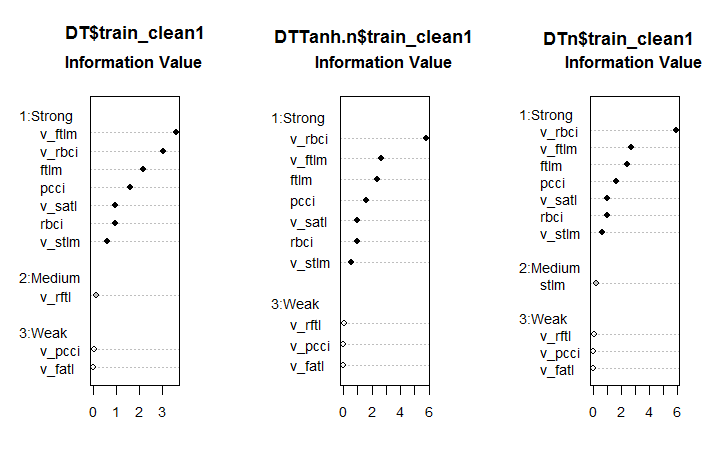NoiseFilterR 软件包含有十几个用于识别噪声样本的其它滤波器。实验快乐！

### 2. 降维

• 它在数据处理期间降低了计算成本
• 减少重复训练的需求。特征越少, 数据中隐藏关系的可靠恢复所需的对象越少, 恢复这种关系的质量越好。
• 压缩数据以更有效地存储信息。在这种情况下, 随同 X → T 变换, 必须有机会进行逆变换 T → X
• 数据可视化。将样本投影到二维或三维空间能够以图形方式表示样本
• 提取新特征。在 X→ T 变换期间获得的新特征也许在以下识别解决方案的实现 (例如主分量分析) 期间可能产生重大影响，

### 2.1. PCA, 主分量分析

(主分量分析, PCA) 是降低数据维度的最简单方法。这种方法的主要思想可追溯到 19 世纪。该方法的原理是在初始空间中找到设置维度的超平面, 并将数据集合投影到此超平面。选择投影数据误差最小的超平面 (方差之和)。

### 2.2. ICA, 独立分量分析

PCA 和 ICA 方法通过 preProcess() 函数在 caret 软件包中实现。在此函数中, 可以设置 PCA 覆盖的主分量数量或累积百分比。独立分量分析有两个阶段。首先, 在训练数据集合上计算所需参数。然后, 使用 predict() 转换数据集合和随后的所有新数据。

方法优点
缺点特点
大多数情况下获得的结果
计算新的主分量的方法
PCA 简单性

• 线性变换
• 对异常值敏感度高
• 不接受 NA
解的非唯一性 (旋转不确定性)。每次基于一次训练测试的新计算都会产生不同的主分量。

• Т — 维度为 [I x A] 的分值矩阵
• P — 维度为 [J x A] 的负载矩阵。从 Х[ ,J] 空间转换矩阵到 PCA [ ,A]
• E — 维度为 [I x J] 的余数矩阵
Tnew = Xnew * P
ICA

• 数据的强制性标准化
• 线性变换
• 对异常值敏感度高
• 不接受 NA
• 适用的分量数量 2-5

• W — 分割矩阵
• K — 预白化矩阵
Snew =scale( Xnew )* W * K

```> ls(env)
 "cap1"         "cap2"         "Close"        "Data"         "dataSet"
 "dataSetCap"   "dataSetClean" "dataSetOut"   "High"         "i"
 "k"            "k.cap"        "k.out"        "lof.x"        "lof.x.cap"
 "Low"          "lower"        "med"          "Open"         "out.ftlm"
 "out.ftlm1"    "pr"           "pre.outl"     "preProClean"  "Rlof.x"
 "Rlof.x.cap"   "sk"           "sk.cap"       "sk.out"       "test"
 "test.out"     "train"        "train.out"    "upper"        "Volume"
 "x"            "x.cap"        "x.out"

```

```require(caret)
evalq({
prePCA <- preProcess(x.cap,
pcaComp = 5,
method = Hmisc::Cs(center, scale, pca))
preICA <- preProcess(x.cap,
n.comp = 3,
method = "ica")
}, env)

```

```> str(env\$prePCA)
List of 20
\$ dim              : int [1:2] 7906 11
\$ bc               : NULL
\$ yj               : NULL
\$ et               : NULL
\$ invHyperbolicSine: NULL
\$ mean             : Named num [1:11] -0.001042 -0.003567 -0.000155 -0.000104 -0.000267 ...
..- attr(*, "names")= chr [1:11] "ftlm" "stlm" "rbci" "pcci" ...
\$ std              : Named num [1:11] 0.091 0.237 0.1023 0.0377 0.0356 ...
..- attr(*, "names")= chr [1:11] "ftlm" "stlm" "rbci" "pcci" ...
\$ ranges           : NULL
\$ rotation         : num [1:11, 1:5] -0.428 -0.091 -0.437 -0.107 -0.32 ...
..- attr(*, "dimnames")=List of 2
.. ..\$ : chr [1:11] "ftlm" "stlm" "rbci" "pcci" ...
.. ..\$ : chr [1:5] "PC1" "PC2" "PC3" "PC4" ...
\$ method           :List of 4
..\$ center: chr [1:11] "ftlm" "stlm" "rbci" "pcci" ...
..\$ scale : chr [1:11] "ftlm" "stlm" "rbci" "pcci" ...
..\$ pca   : chr [1:11] "ftlm" "stlm" "rbci" "pcci" ...
..\$ ignore: chr(0)
\$ thresh           : num 0.95
\$ pcaComp          : num 5
\$ numComp          : num 5
\$ ica              : NULL
\$ wildcards        :List of 2
..\$ PCA: chr(0)
..\$ ICA: chr(0)
\$ k                : num 5
\$ knnSummary       :function (x, ...)
\$ bagImp           : NULL
\$ median           : NULL
\$ data             : NULL
- attr(*, "class")= chr "preProcess"

```

```> str(env\$preICA)
List of 20
\$ dim              : int [1:2] 7906 11
\$ bc               : NULL
\$ yj               : NULL
\$ et               : NULL
\$ invHyperbolicSine: NULL
\$ mean             : Named num [1:11] -0.001042 -0.003567 -0.000155 -0.000104 -0.000267 ...
..- attr(*, "names")= chr [1:11] "ftlm" "stlm" "rbci" "pcci" ...
\$ std              : Named num [1:11] 0.091 0.237 0.1023 0.0377 0.0356 ...
..- attr(*, "names")= chr [1:11] "ftlm" "stlm" "rbci" "pcci" ...
\$ ranges           : NULL
\$ rotation         : NULL
\$ method           :List of 4
..\$ ica   : chr [1:11] "ftlm" "stlm" "rbci" "pcci" ...
..\$ center: chr [1:11] "ftlm" "stlm" "rbci" "pcci" ...
..\$ scale : chr [1:11] "ftlm" "stlm" "rbci" "pcci" ...
..\$ ignore: chr(0)
\$ thresh           : num 0.95
\$ pcaComp          : NULL
\$ numComp          : NULL
\$ ica              :List of 3
..\$ row.norm: logi FALSE
..\$ K       : num [1:11, 1:3] -0.214 -0.0455 -0.2185 -0.0534 -0.1604 ...
..\$ W       : num [1:3, 1:3] -0.587 0.77 -0.25 -0.734 -0.636 ...
\$ wildcards        :List of 2
..\$ PCA: chr(0)
..\$ ICA: chr(0)
\$ k                : num 5
\$ knnSummary       :function (x, ...)
\$ bagImp           : NULL
\$ median           : NULL
\$ data             : NULL
- attr(*, "class")= chr "preProcess"

```

• 初始矩阵居中 (可能是行规范化), 我们得到 Х 矩阵
• 然后 Х 矩阵被投影到主分量的方向, 我们得到 PCA = X * K 作为结果
• 之后, PCA 乘以混合矩阵, 得到独立分量 ICA = X * K * W

```require(magrittr)
evalq({
pca <- predict(prePCA, x.cap)
ica <- predict(preICA, x.cap)
pca1 <- ((x.cap %>% scale(prePCA\$mean, prePCA\$std)) %*%
prePCA\$rotation)
ica1 <- ((x.cap %>% scale(preICA\$mean, preICA\$std)) %*%
preICA\$ica\$K) %*% preICA\$ica\$W
colnames(ica1) <- colnames(ica1, do.NULL = FALSE, prefix = 'ICA')

},env)
evalq(all_equal(pca, pca1), env)
#  TRUE
evalq(all_equal(ica, ica1), env)
#  TRUE

```

fastICA 软件包含有大量附加函数和参数, 可以更灵活, 更完整地计算独立分量。我建议使用 fastICA, 而不是 caret 中的函数。

### 2.3概率主分量分析 (PPCA)

PCA 有一个概率模型 - PPCA。以概率术语重新定义此方法具有许多优点。

• 有机会使用 ЕМ 算法来搜索解决方案。当 d ≪ D 的情形下, ЕМ 算法是一种更有效的计算过程
• 正确处理缺失值。它们被简单地添加到概率模型的隐藏变量列表中。然后将 ЕМ 算法应用于该模型。
• 有过渡到混合物分布模型的可能性, 拓宽了该方法的适用性
• 使用贝叶斯方法解决选择模型问题的可能性。特别地, 可以构建约化空间维度 d 的理论上合理的选择方案 (参见 [24,25])
• 从概率模型生成新对象的可能性
• 为了分类起见 - 单个对象类模型分布的可能性, 以便进一步在分类方案中使用
• likelihood 函数的值是一个通用准则, 允许不同的概率模型相互比较。具体来说, 可以使用 likelihood 值轻易地识别数据集合中的异常值。

pcaMethods 软件包是一套 PCA 的各种实现, 以及用于交叉验证和结果可视化的工具。这些方法主要允许将 PCA 应用于不完整的数据集合, 因此可用于评估缺失值 (NA)。软件包可在储存库 Bioconductor 中找到, 可以按照下面的说明进行安装。

```source("https://bioconductor.org/biocLite.R")
biocLite("pcaMethods")
library(pcaMethods)

```

• svdPCA 是标准函数 prcomp 的封装函数。
• svdImpute 是插补 NA 的算法实现。这对于大量 NA (>10%) 是宽容的。
• 概率 PCA (ppca)。PPCA 容忍的 NA 数量在 10％ 至 15％之间。
• 贝叶斯 PCA (bpca)。类似于概率 PCA, 它使用 EM 方法与贝叶斯模型来计算恢复值的概率。此算法容忍相对较大数量的缺失数据 (>10％)。通过该方法获得的分值和负载与使用普通的 РСА 获得的分值和负载不同。事实上, BPCA 是 专为评估缺失值 而开发的, 且基于具有自动相关性识别 (ARD) 的变异贝叶斯框架 (VBF)。该算法不在负载之间强制正交性。事实上, BPCA 的作者发现包括正交准则令预测变得更糟。
• 逆向非线性 PCA (NLPCA) 最适合于预测因子与目标变量之间的关系是非线性的实验数据。NLPCA 基于关联神经网络 (autoencoder) 的解码部分的训练。可以在网络的隐藏层看到负载。在反向传播过程中, 误差计算忽略训练数据中的缺失值。这样, NLPCA 可以用于处理缺失值, 与标准的 РСА 相同。唯一的区别是现在由负载 P 代表神经网络。我们将在 "Autoencoder" 部分中更详细地研究这种降维方式。
• Nipals PCA 是通过迭代偏最小二乘法的非线性评估。这是 PLS 回归的一种核心算法, 它可以执行缺失值的 PCA, 将它们遗留在相应内部产品之外。它容忍少量 (通常不超过 5％) 的缺失数据。
• 局部最小二乘法 (LLS) 插补llsImpute() 的算法/函数, 基于不完整变量的 k-最相邻线性组合评估缺失数据。变量之间的距离定义为 Pearson, Spearman 或 Kendall 相关系数的绝对值。最优线性组合可以通过求解局部最小二乘法问题找到。

### 2.4. Autoencoder

Autoencoder 是具有一个或多个隐藏层的神经网络, 且输入层中的神经元数量等于输出层中神经元的数量。АЕ 的主要目的是尽可能准确地表示输入数据。用于标准神经网络训练的正则化和神经元激活方法也同样用于 AE。任何构建神经网络的软件包只要允许提取隐藏层权重矩阵, 均可用来构建 AE 模型, 。我们将使用 autoencoder 软件包。以下示例将有助于回顾可能的 AE 结构: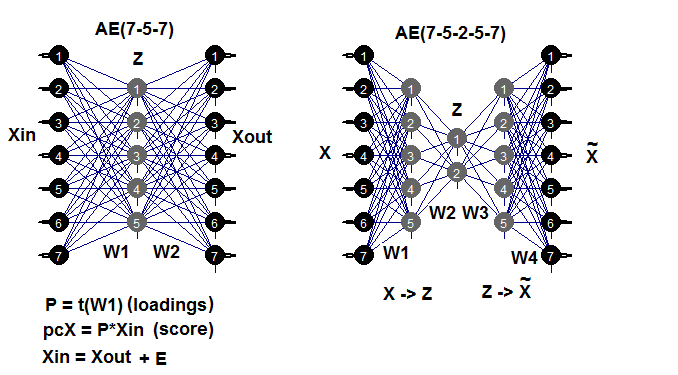• 从 dataSet 集合中创建训练/验证/测试数据集合, 并获取 DT 列表;
• 插补异常值并获取 DTcap 列表;
• 使用带 ("center", "scale", "spatialSign") 的方法将我们的集合进行常规化。您可以使用我们以前研究的其它变换和常规化方法;
• 在隐藏层中用三个神经元训练 autoencoder 模型。您可以探索其它变种。随着神经元数量的增加, 恢复误差降低;
• 使用已训练模型和 predict(), 从隐藏层获得结果。这基本上是一个约化矩阵 (РСА)。向其添加目标变量;
• 绘制变体和训练/验证测试约化样本协方差的图表。
```require(FCNN4R)
require(deepnet)
require(darch)
require(tidyverse)
require(magrittr)
#----清理---------------------
require(caret)
require(pipeR)
evalq(
{
train = 1:2000
val = 2001:3000
test = 3001:4000
DT <- list()
dataSet %>%
preProcess(., method = c("zv", "nzv", "conditionalX")) %>%
predict(., dataSet) %>%
na.omit -> dataSetClean
list(train = dataSetClean[train, ],
val = dataSetClean[val, ],
test = dataSetClean[test, ]) -> DT
rm(dataSetClean, train, val, test)
},
env)
#------异常值-------------
require(foreach)
evalq({
DTcap <- list()
foreach(i = 1:3) %do% {
DT[[i]] %>%
select(-c(Data, Class)) %>%
as.data.frame() -> x
if (i == 1) {
foreach(i = 1:ncol(x), .combine = "cbind") %do% {
prep.outlier(x[ ,i]) %>% unlist()
} -> pre.outl
colnames(pre.outl) <- colnames(x)
}
foreach(i = 1:ncol(x), .combine = "cbind") %do% {
stopifnot(exists("pre.outl", envir = env))
lower = pre.outl['lower.25%', i]
upper = pre.outl['upper.75%', i]
med = pre.outl['med', i]
cap1 = pre.outl['cap1.5%', i]
cap2 = pre.outl['cap2.95%', i]
treatOutlier(x = x[ ,i], impute = T, fill = T,
lower = lower, upper = upper,
med = med, cap1 = cap1, cap2 = cap2)
} %>% as.data.frame() -> x.cap
colnames(x.cap) <- colnames(x)
return(x.cap)
} -> DTcap
foreach(i = 1:3) %do% {
cbind(DTcap[[i]], Class = DT[[i]]\$Class)
} -> DTcap
DTcap\$train <- DTcap[]
DTcap\$val <- DTcap[]
DTcap\$test <- DTcap[]
rm(lower, upper, med, cap1, cap2, x.cap, x)
}, env)
#------常规化-----------
evalq(
{
method <- c("center", "scale", "spatialSign") #, "expoTrans") #"YeoJohnson",
# "spatialSign"
preProcess(DTcap\$train, method = method) -> preproc
list(train = predict(preproc, DTcap\$train),
val = predict(preproc, DTcap\$val),
test = predict(preproc, DTcap\$test)
) -> DTcap.n
#foreach(i = 1:3) %do% {
#  cbind(DTcap.n[[i]], Class = DT[[i]]\$Class)
#} -> DTcap.n
},
env)
#----训练-------
require(autoencoder)
evalq({
train <-  DTcap.n\$train %>% select(-Class) %>% as.matrix()
val <-  DTcap.n\$val %>% select(-Class) %>% as.matrix()
test <-  DTcap.n\$test %>% select(-Class) %>% as.matrix()
## 设置 autoencoder 架构:
nl = 3                    ## number of layers (default is 3: input, hidden, output)
unit.type = "tanh"        ## specify the network unit type, i.e., the unit's
## 激活函数 ("logistic" 或 "tanh")
N.input = ncol(train)   ## 输 层中的单位数 (每像素一个单位)
N.hidden = 3              ## 隐藏层中的单位数
lambda = 0.0002           ## 重量衰减参数
beta = 0                  ## 稀疏项权重系数
rho = 0.01                ## 期望的稀疏参数
epsilon <- 0.001          ## 用于初始化权重的小参数
## 来自 N(0,epsilon^2) 的样本作为小高斯随机数
max.iterations = 3000     ## 优化器中的迭代次数
## 使用 BFGS 训练 autoencoder
## 优化方法
AE_13 <- autoencode(X.train = train, X.test = val,
nl = nl, N.hidden = N.hidden,
unit.type = unit.type,
lambda = lambda,
beta = beta,
rho = rho,
epsilon = epsilon,
optim.method = "BFGS", #"BFGS", "L-BFGS-B", "CG"
max.iterations = max.iterations,
rescale.flag = FALSE,
rescaling.offset = 0.001)}, env)
## 训练和测试集合的平均方差报告:
#cat("autoencode(): mean squared error for training set: ",
#    round(env\$AE_13\$mean.error.training.set,3),"\n")
## 从 autoencoder.object 提取权重 W 和偏差 b:
#evalq(P <- AE_13\$W, env)
#-----predict-----------
evalq({
#Train <- predict(AE_13, X.input = train, hidden.output = FALSE)
pcTrain <- predict(AE_13, X.input = train, hidden.output = TRUE)\$X.output %>%
tbl_df %>% cbind(., Class = DTcap.n\$train\$Class)
#Val <- predict(AE_13, X.input = val, hidden.output = FALSE)
pcVal <- predict(AE_13, X.input = val, hidden.output = TRUE)\$X.output %>%
tbl_df %>% cbind(., Class = DTcap.n\$val\$Class)
#Test <- predict(AE_13, X.input = test, hidden.output = FALSE)
pcTest <- predict(AE_13, X.input = test, hidden.output = TRUE)\$X.output %>%
tbl_df %>% cbind(., Class = DTcap.n\$test\$Class)
}, env)
#-----图形---------------
require(GGally)
evalq({
ggpairs(pcTrain,columns = 1:ncol(pcTrain),
mapping = aes(color = Class),
title = "pcTrain")},
env)
evalq({
ggpairs(pcVal,columns = 1:ncol(pcVal),
mapping = aes(color = Class),
title = "pcVal")},
env)
evalq({
ggpairs(pcTest,columns = 1:ncol(pcTest),
mapping = aes(color = Class),
title = "pcTest")},
env)

```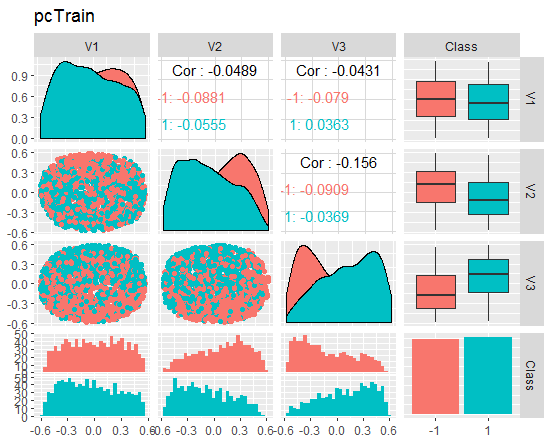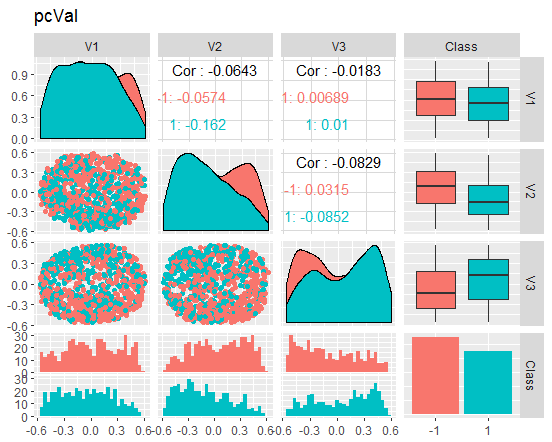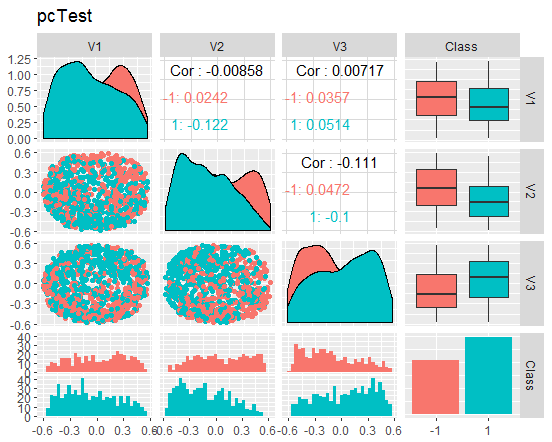### 2.5. 逆向非线性 PCA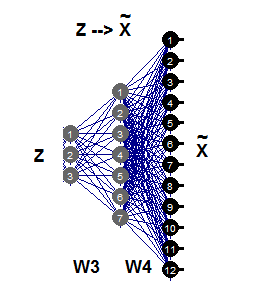```require(pcaMethods)
evalq({
DTcap.n\$train %>% tbl_df %>%
select(-Class) %>% as.matrix() %>%
prep(scale = "none", center = TRUE) -> train
resNLPCA <- pca(train,
method = "nlpca", weightDecay = 0.01,
unitsPerLayer = c(3, 8, 12),
center = TRUE, scale = "none",# c("none", "pareto", "vector", "uv")
nPcs = 3, completeObs = FALSE,
subset = NULL, cv = "none", # "none""q2"), ...)
maxSteps = 1100)
rm(train)},
env)
#--------
evalq(
pcTrain <- resNLPCA@scores %>% tbl_df %>%
cbind(., Class = DTcap.n\$train\$Class)
, env)
#------graph-------
require(GGally)
evalq({
ggpairs(pcTrain,columns = 1:ncol(pcTrain),
mapping = aes(color = Class),
title = "pcTrain -> NLPCA(3-8-12) wd = 0.01")},
env)
#----------

```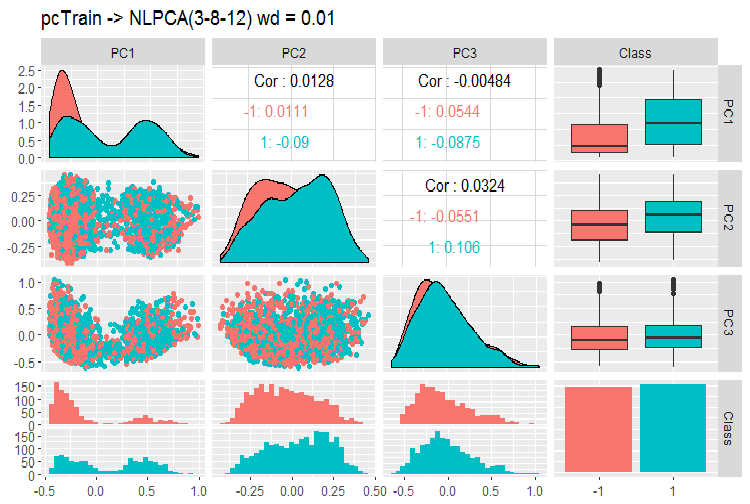```> print(env\$resNLPCA)
nlpca calculated PCA
Importance of component(s):
PC1    PC2     PC3
R2            0.3769 0.2718 0.09731
Cumulative R2 0.3769 0.6487 0.74599
12      Variables
2000    Samples
0       NAs ( 0 %)
3       Calculated component(s)
Data was mean centered before running PCA
Data was NOT scaled before running PCA
Scores structure:
 2000    3
Inverse hierarchical neural network architecture
3 8 12
Functions in layers
linr tanh linr
hierarchic layer: 1
hierarchic coefficients: 1 1 1 0.01
scaling factor: 0.3260982

```

```#=======PPCA===================
evalq({
DTcap.n\$train %>% tbl_df %>%
select(-Class) %>% as.matrix() -> train
DTcap.n\$val %>% tbl_df %>%
select(-Class) %>% as.matrix() -> val
DTcap.n\$test %>% tbl_df %>%
select(-Class) %>% as.matrix() -> test
resPPCA <- pca(train, method = "ppca",
center = TRUE, scale = "none",# c("none", "pareto", "vector", "uv")
nPcs = 3, completeObs = FALSE,
subset = NULL, cv = "none", # "none""q2"), ...)
maxIterations = 3000)
},
env)
#-----------
>print(env\$resPPCA)
ppca calculated PCA
Importance of component(s):
PC1 PC2 PC3
R2 0.2873 0.2499 0.1881
Cumulative R2 0.2873 0.5372 0.7253
12 	Variables
2000 	Samples
0 	NAs ( 0 %)
3 	Calculated component(s)
Data was mean centered before running PCA
Data was NOT scaled before running PCA
Scores structure:
 2000 3
 12 3

```

```slplot(env\$resPPCA, pcs = c(1,2),
lcex = 0.9, sub = "Probabilistic PCA")

```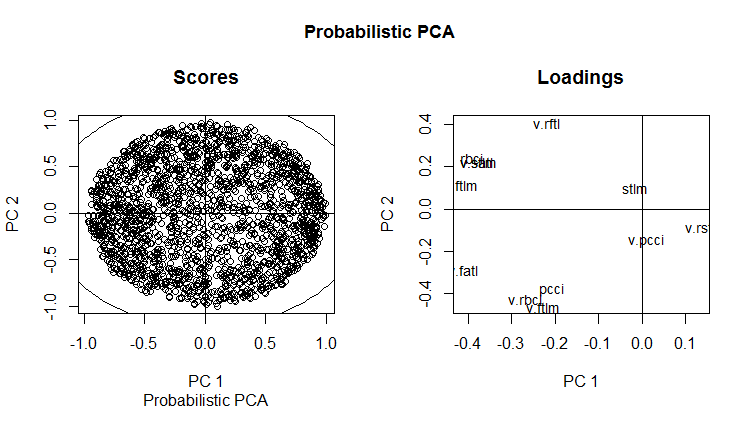PPCA 训练/验证/测试集合的主分量的变体和协方差图表如下所示。您可在 GitHub 上找到脚本。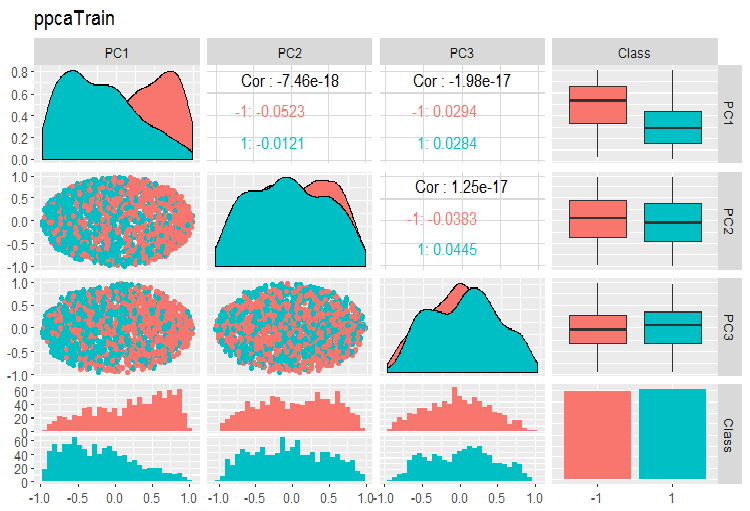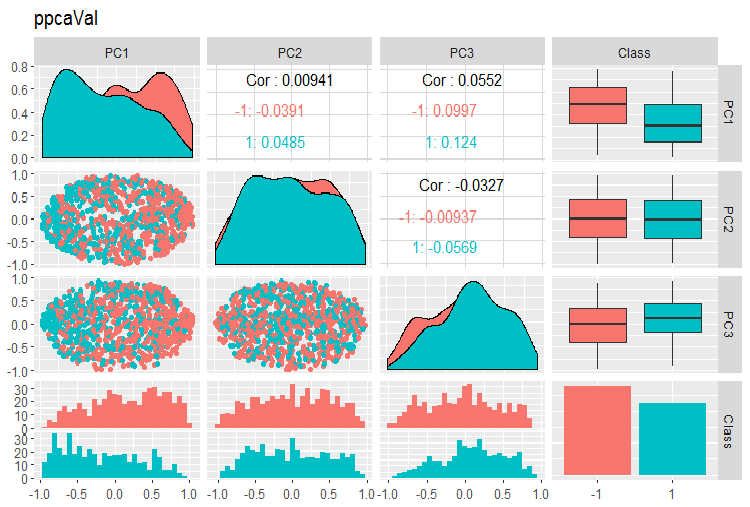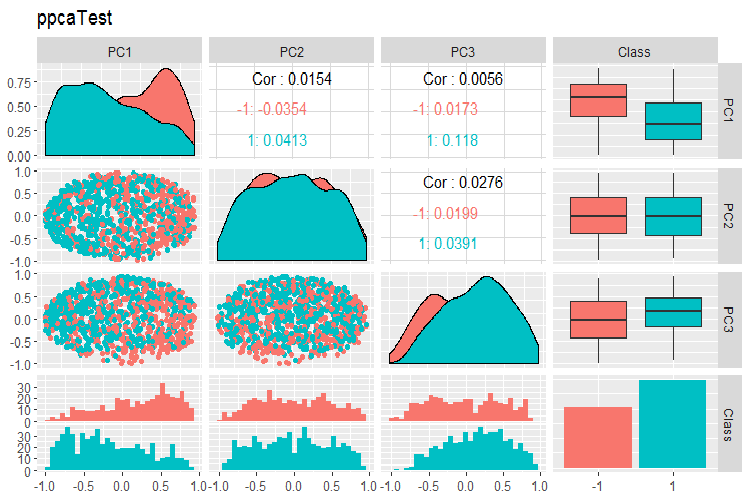### 应用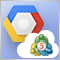使用云存储服务来进行终端之间的数据交换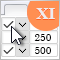图形界面 XI: 表格单元中的文本编辑框和组合框 (统合构建15)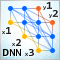深度神经网络 (第 IV 部)。创建, 训练和测试神经网络模型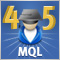跨平台智能交易系统: 停止位